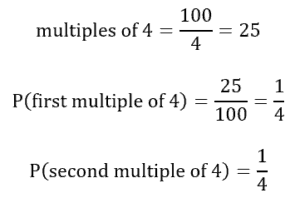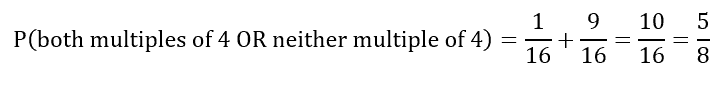# PCAT Quantitative Reasoning – Probability of Random Number Selection

When randomly selecting two numbers between 1 and 100, what is the probability that both will be a multiple of 4 or neither will be a multiple of 4? The same number can be chosen twice.

A. 3/16
B. 5/16
C. 3/8
D. 5/8

##### Click for Explanation

D is correct. First, let’s identify the probability of selecting two multiples of 4 in a row. The number of multiples of four (4, 8, 12, etc.) is equal to:Recall that the same number can be selected twice! The probability of both events occurring is equal to the product of their probabilities:Now let’s determine the probability of neither being a multiple of 4:The probability of the first situation occurring or the second situation occurring is the sum of their probabilities:#### Submit a Comment

This site uses Akismet to reduce spam. Learn how your comment data is processed.